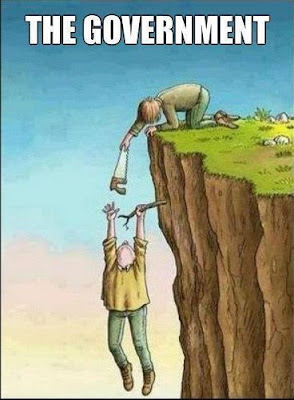## Sunday, February 10, 2019

### Tesla's Fascination with 3 6 9

“Philosophy [nature] is written in that great book which ever is before our eyes (I mean the universe) but we cannot understand it if we do not first learn the language and grasp the symbols in which it is written. The book is written in mathematical language, and the symbols are triangles, circles and other geometrical figures, without whose help it is impossible to comprehend a single word of it; without which one wanders in vain through a dark labyrinth.” Galileo Galilei

Nikola Tesla on 3, 6 and 9 :
If you only knew the magnificence of the 3, 6 and 9 then you would have a key to the universe.

The 3, 6 and 9 represent the pathways that force (energy / information) manifest through to form material.

The other number 1 , 2 , 4 , 5 , 7 , 8 represent the already manifested ingredients of material.

Thus the structure of all things material is dependent on the sequence / pattern force takes via the 3 , 6 and 9 conduits

The sequence changes to form the pulses (wave) force found forming and supporting all things commonly known as fields.

Since 9 is 3 squared and 3 is the first prime. (Squared = enclosing space = a complete 360 degrees). Everything contained here in will be expressing this “3” or third principal. Space is defined by 3 dimensions. When working with these number patterns, always think in terms of thirds.
Now it becomes somewhat clearer as to why there are 360 degrees in a circle. They are all expressing decimal parity of 9 which is to say; centre focused. Even if you halve 45 and so on you will get 22.5 = 9, 11.25 = 9, 5.625, 2.8125 = 9 and so on. The 9 is a self similar axis, the Z axis. The X and Y define the surface topology of the logarithmic spiral, while Z is the central third. 6 and 3 oscillating around nine and it’s probably 360 because 3 and 6 are the “nines”. So that’s 3 dimensional spatial orientation.

Earth Latitude and Longitude in Degrees (3 6 9)

4th dimension; time:

There are 24 hours in a day; 2+4 = 6

There are 60 minutes in an hour; 6+0 = 6

There are 60 second in a minute; 6+0 = 6

So the full revolution is a 6, half is 12 which is a 3. Around the 12 hour clock you constant 3,6,9 oscillation. 3oclock, 6oclock, 9oclock, 12oclock= 3 then 15 = 6, 18 = 9.... 3 , 6 , 9 , 3 , 6 , 9 , 3 ,6 (8 45 degree movement)

Numbers have temporal (series of values over a time period), spatial (occupying) and volumetric qualities. Space and time are one thing; “space-time”. They are both spirals. Everything is a curved line except the 9; the spirit emanation outside of space and time.

Not only that but an atom is 99.9999 % space; it is a mini blackhole. Electromagnetism is radiating out in exchange of gravity going into the 9.

The 9 demonstrates the omni dimension which is the higher dimensional flux emanation called Spirit that always occurs within the center of the magnetic field lines.

The number nine is Energy being manifested in a single moment event of occurrence in our physical world of creation. It is unique because it is the focal center by being the only number identifying with the vertical upright axis.

It is the singularity or the Primal Point of Unity. The number nine never changes and is linear. For example all multiples of 9 equal 9.

9 x 1 = 9, 9 x 2 = 18, but 1 + 8 = 9, 9 x 3 = 27, but 2 + 7 = 9. This is because it is emanating in a straight line from the center of mass out of the nucleus of every atom, and from out of the singularity of a black hole. It is complete, revealing perfection, and has no parity because it always equals itself.

This is because it is flowing in a straight line from the center of mass out of the nucleus of every atom, and from out of the singularity of a black hole. It is complete, revealing perfection and has no parity because it always equals itself.

The number nine lines up with the center of the infinity symbol and it is from this center that the linear emanations we call Spirit flow from the center of mass outwards. Spirit is the only thing in the universe that moves in a straight line. Spirit is what makes everything else warp and curve around it. The perfect number patterns are actually created by this Spirit energy. Without Spirit the universe would become destitute and void. Spirit flow is the source of all movement as well as the source of the non-decaying spin of the electron.
Notice how the 3, 9 and 6 are in red and do not connect at the base. That is because it is a vector. The 1 , 2 , 4 , 8 , 7 and 5 is the third dimension while the oscillation between the 3 and 6 demonstrates the fourth dimension, which is the higher dimensional magnetic field of an electrical coil.

The 3, 9 and 6 always occur together with the 9 as the control. In fact, the Yin / Yang is not a duality but rather a trinary. This is because the 3 and 6 represent each side of the Yin / Yang and the 9 is the "S" curve between them. Everything is based on thirds. We think that the universe is based on dualities because we see the effects not the cause.